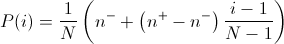io.jenetics

## Class LinearRankSelector<G extends Gene<?,G>,C extends Comparable<? super C>>

• All Implemented Interfaces:
Selector<G,C>

public final class LinearRankSelector<G extends Gene<?,G>,C extends Comparable<? super C>>
extends ProbabilitySelector<G,C>

In linear-ranking selection the individuals are sorted according to their fitness values. The rank N is assignee to the best individual and the rank 1 to the worst individual. The selection probability P(i) of individual i is linearly assigned to the individuals according to their rank.Here n-/N is the probability of the worst individual to be selected and n+/N the probability of the best individual to be selected. As the population size is held constant, the conditions n+ = 2 - n- and n- >= 0 must be fulfilled. Note that all individuals get a different rank, i.e., a different selection probability, even if the have the same fitness value.

T. Blickle, L. Thiele, A comparison of selection schemes used in evolutionary algorithms, Technical Report, ETH Zurich, 1997, page 37. http://citeseer.ist.psu.edu/blickle97comparison.html

Since:
1.0
Version:
5.0
Author:
Franz Wilhelmstötter

• ### Fields inherited from class io.jenetics.ProbabilitySelector

_reverter, _sorted, POPULATION_COMPARATOR
• ### Constructor Summary

Constructors
Constructor and Description
LinearRankSelector()
Create a new LinearRankSelector with nminus := 0.5.
LinearRankSelector(double nminus)
Create a new LinearRankSelector with the given values for nminus.
• ### Method Summary

All Methods
Modifier and Type Method and Description
protected double[] probabilities(Seq<Phenotype<G,C>> population, int count)
This method sorts the population in descending order while calculating the selection probabilities.
String toString()
• ### Methods inherited from class io.jenetics.ProbabilitySelector

probabilities, select
• ### Methods inherited from class java.lang.Object

clone, equals, finalize, getClass, hashCode, notify, notifyAll, wait, wait, wait
• ### Constructor Detail

• #### LinearRankSelector

public LinearRankSelector(double nminus)
Create a new LinearRankSelector with the given values for nminus.
Parameters:
nminus - nminus/N is the probability of the worst phenotype to be selected.
Throws:
IllegalArgumentException - if nminus < 0.
• #### LinearRankSelector

public LinearRankSelector()
Create a new LinearRankSelector with nminus := 0.5.
• ### Method Detail

• #### probabilities

protected double[] probabilities(Seq<Phenotype<G,C>> population,
int count)
This method sorts the population in descending order while calculating the selection probabilities.
Specified by:
probabilities in class ProbabilitySelector<G extends Gene<?,G>,C extends Comparable<? super C>>
Parameters:
population - The unsorted population.
count - The number of phenotypes to select. This parameter is not needed for most implementations.
Returns:
Probability array. The returned probability array must have the length population.size() and must sum to one. The returned value is checked with assert(Math.abs(math.sum(probabilities) - 1.0) < 0.0001) in the base class.
• #### toString

public String toString()
Overrides:
toString in class Object

© 2007-2019 Franz Wilhelmstötter  (2019-11-18 20:30)